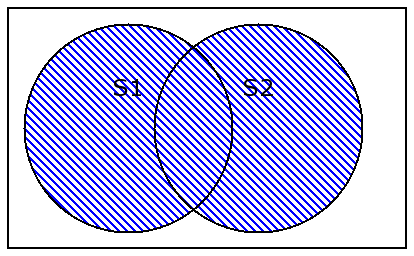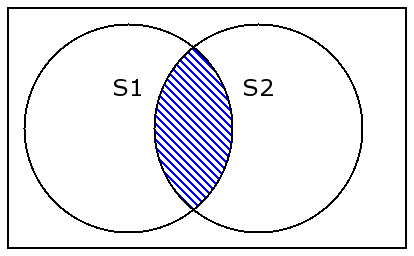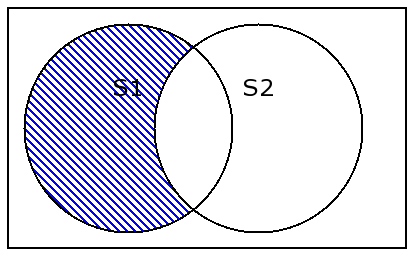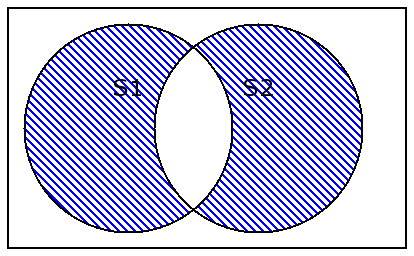On-line GuidesAll GuideseBook StoreiOS / AndroidLinux for BeginnersOffice ProductivityLinux InstallationLinux SecurityLinux UtilitiesLinux VirtualizationLinux KernelSystem/Network AdminProgrammingScripting LanguagesDevelopment ToolsWeb DevelopmentGUI Toolkits/DesktopDatabasesMail SystemsopenSolarisEclipse DocumentationTechotopia.comVirtuatopia.comHow To GuidesVirtualizationGeneral System AdminLinux SecurityLinux FilesystemsWeb ServersGraphics & DesktopPC HardwareWindowsProblem SolutionsPrivacy Policy## Set Operations

There are a large number of `set` operations, including union (`|`), intersection (`&`), difference (`-`), symmetric difference (`^`). These are unusual operations, so we'll look at them in some detail. In addition to this operator notation, there are method functions which do the same things. We'll look at the method function versions below.

We'll use the following two `set`s to show these operators.

````>>>`
`fib=set( (1,1,2,3,5,8,13) )`

`>>>`
`prime=set( (2,3,5,7,11,13) )`

```
Union, |

The resulting `set` has elements from both source `set`s. An element is in the result if it is one `set` or the other.

````>>>`
`fib | prime`

`set([1, 2, 3, 5, 7, 8, 11, 13])`
```

Figure 16.1. Set Union, S1|S2Intersection, &

The resulting `set` has elements that are common to both source `set`s. An element is in the result if it is in one `set` and the other.

````>>>`
`fib & prime`

`set([2, 3, 5, 13])`
```

Figure 16.2. Set Intersection, S1&S2Difference, -

The resulting `set` has elements of the left-hand `set` with all elements from the right-hand `set` removed. An element will be in the result if it is in the left-hand `set` and not in the right-hand `set`.

````>>>` `fib - prime`
`set([8, 1])`
`>>>` `prime - fib`
`set([11, 7])`
```

Figure 16.3. Set Difference, S1-S2Symmetric Difference, ^

The resulting `set` has elements which are unique to each `set`. An element will be in the result `set` if either it is in the left-hand `set` and not in the right-hand `set` or it is in the right-hand `set` and not in the left-hand `set`. Whew!

````>>>`
`fib ^ prime`

`set([8, 1, 11, 7])`
```

Figure 16.4. Set Symmetric Difference, S2^S2Published under the terms of the Open Publication License Design by Interspire